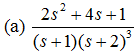## Determine standard deviation, MATLAB Programming

Assignment Help:

Find f(t) for each F(s):Problem:

Based on an examination given to a large class in which the maximum score is 100 points, assuming that 20 grades taken at random from the entire set produced the results that follow:

43 49 56 61 65 68 70 70 74 74 76 79 80 83 84 87 88 92 96 100

Determine analytically the:

a sample mean

b sample mean-square

c sample rms value

d sample variance

e sample standard deviation

f median

g mode

h range

e compute all the above values using Matlab.

#### Structure tensor, How to calculate 2D/3D structure tensor of image

How to calculate 2D/3D structure tensor of image

#### Homework, -The program should run always until the user enters -1 to exit f...

-The program should run always until the user enters -1 to exit from the program. - In the main, you should ask user to input any angles in degrees (Ad) and an integer number (N).

#### Matlab code using ant colony algorithm, Ant Colony Optimization for Optimiz...

Ant Colony Optimization for Optimization of Route path taken Ant Colony Algorithm is a potential candidate for coupled, 3D optimisation. Ant Colony Algorithm enjoys the overall

#### Filter design, IIR filter using Bilinear trasformation

IIR filter using Bilinear trasformation

#### If-else statement, The IF-ELSE Statement: The if statement selects whe...

The IF-ELSE Statement: The if statement selects whether an action is executed or not. Selecting between the two actions, or selecting from numerous actions, is accomplished by

#### Looping statements, Looping Statements: Consider the problem of comput...

Looping Statements: Consider the problem of computing the area of a circle with radius of 0.3 centimeters-a MATLAB program certainly is not required to do that; you would use

construction

#### Function definitions, Function definitions: There are various ways to ...

Function definitions: There are various ways to organize the scripts and functions, but for now every function which we write will be stored in a separate M-file, that is why

#### Crank-nicolson method, clear tic L=1; T=0.2; nust=2000; dt=T...

clear tic L=1; T=0.2; nust=2000; dt=T/nust; n=40; dx=L/n;   r=1;  omega=10:10:5000;%Store Range of Frequencies for Simulation u=zeros(n+1,nust+1);%

#### Characters and encoding, Characters and Encoding: A character in a MAT...

Characters and Encoding: A character in a MATLAB is represented by using single quotes (example, 'a' or 'x'). The quotes are essential to denote a character; without them, the